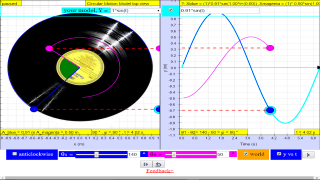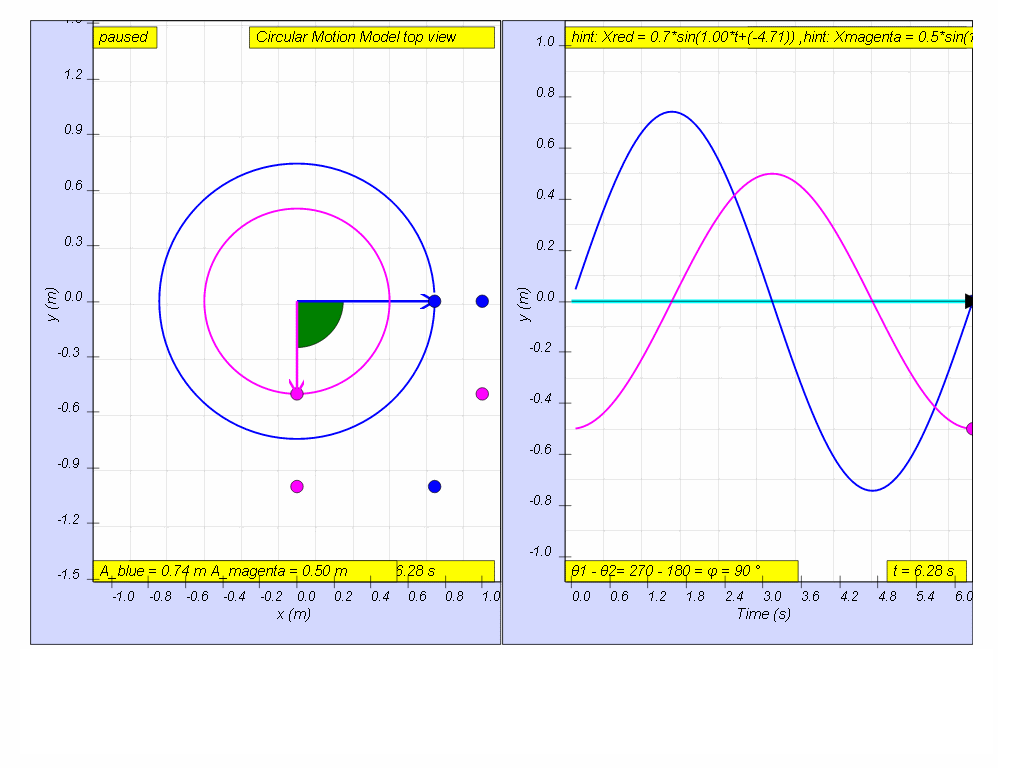Example 3: Uniform Circular motion’s one dimensional projection

### 1.1.3.2.3 Understanding Phase Difference through Uniform Circular Motion

Simple harmonic motion can in some cases be considered to be the one-dimensional projection of uniform circular motion. If an object moves with angular speed ω around a circle of radius A centered at the origin of the x−y plane, then its motion along each coordinate is simple harmonic motion with amplitude A and angular frequency ω.## Q1: given that, a circular motion can be described by x = A cos(ω t)  and y A sin(ω t) what is the y-component model-equation that can describe the motion of a uniform circular motion?

A1: y = Asin (ωt)

## Q2: When the x-component of the circular motion is modelled by x = A cos(ω t)  and y A sin(ω t) suggest an model-equation for y.

A2: y = Acos (ωt) for top position or y = - Acos (ωt) for bottom position

## Q3: Explain why are the models for both x and y projection of a uniform circular motion, a simple harmonic motion?

A3: both x = A cos(ω t)  and y A sin(ω t) each follow the defining relationship for SHM as ordinary differential equations of   $\frac{{d}^{2}x}{{dt}^{2}}=-{\omega }^{2}x$ and  $\frac{{d}^{2}y}{{dt}^{2}}=-{\omega }^{2}y$ respectively.

## Q4: In, the diagram above, what is the phase difference between blue and magenta color y direction motion?

A4: the phase difference is 90 degrees, or more precisely blue lead red by 90 degrees angle.

http://youtu.be/0IaKcqRw_Ts This video shows how a pendulum's oscillations and the shadow of rotating object are related. This could be used to demonstrate that the projection of a circular motion is actually a simple harmonic motion.

### Translations

Code Language Translator Run### Software Requirements

SoftwareRequirements

 Android iOS Windows MacOS with best with Chrome Chrome Chrome Chrome support full-screen? Yes. Chrome/Opera No. Firefox/ Samsung Internet Not yet Yes Yes cannot work on some mobile browser that don't understand JavaScript such as..... cannot work on Internet Explorer 9 and below

### CreditsThis email address is being protected from spambots. You need JavaScript enabled to view it.; Fu-Kwun Hwang

### end faq

http://iwant2study.org/lookangejss/02_newtonianmechanics_8oscillations/ejss_model_SHM045/SHM045_Simulation.xhtml

[text]

# Testimonials (0)

There are no testimonials available for viewing. Login to deploy the article and be the first to submit your review!